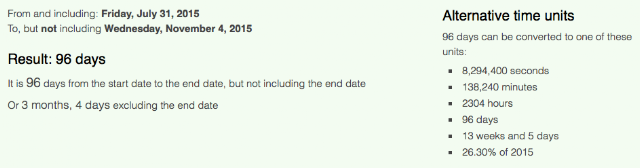## Saturday, October 31, 2015

### 53 76 88 93 | The Date Yoenis Cespedes Was Traded from Tigers to Mets to the World Series

The Mets went down 5-3 in Game 4 of the 111th World Series, in large part, thanks to some sloppy play by Yoenis Cespedes.  Notice the date he was traded from the Tigers to the Mets on.

7/31/15 = 7+31+15 = 53

The World Series began 88-days after the trade.

Tomorrow, 11/1, will be 93-days from the July 31st trade.  Remember, the big numbers in New York are 39, 42, 93 and 111.  11/1 for the 111th World Series, in New York (111)?  We'll see what Yoenis has in store for us.

Should this Series make it to Game 7, it will be 96-days after the trade.Yoenis = 7+6+5+5+9+1 = 33/42
Cespedes = 3+5+1+7+5+4+5+1 = 31/49
Yoenis Cespedes = 64/91

Yoenis = 25+15+5+14+9+19 = 87
Cespedes = 3+5+19+16+5+4+5+19 = 76 (10/31/2015 = 76)
Yoenis Cespedes = 163

Notice how the name Cespedes has Gematria of 76, corresponding with the date of Game 4, where he closed off the game, with a base runner error, creating a double play to end the game.

Cespedes was born on a special date.

10/18/1985 = 10+18+19+85 = 132
10/18/1985 = 10+18+(1+9+8+5) = 51
10/18/1985 = 1+0+1+8+1+9+8+5 = 33
10/18/85 = 10+18+85 = 113

October = 6+3+2+6+2+5+9 = 33